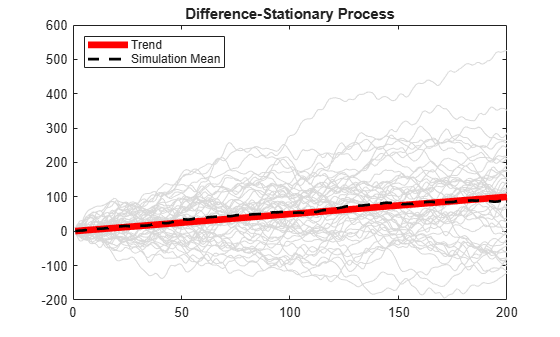# Simulate Trend-Stationary and Difference-Stationary Processes

This example shows how to simulate trend-stationary and difference-stationary processes. The simulation results illustrate the distinction between these two nonstationary process models.

### Generate Observations from Trend-Stationary Process

Specify the trend-stationary process

`${y}_{t}=0.5t+{\epsilon }_{t}+1.4{\epsilon }_{t-1}+0.8{\epsilon }_{t-2},$`

where the innovation process is Gaussian with variance 8. After specifying the model, simulate 50 sample paths of length 200. Use 100 burn-in simulations.

```t = [1:200]'; trend = 0.5*t; MdlTS = arima('Constant',0,'MA',{1.4,0.8},'Variance',8); rng('default') u = simulate(MdlTS,300,'NumPaths',50); Yt = repmat(trend,1,50) + u(101:300,:); figure plot(Yt,'Color',[.85,.85,.85]) hold on h1 = plot(t,trend,'r','LineWidth',5); xlim([0,200]) title('Trend-Stationary Process') h2 = plot(mean(Yt,2),'k--','LineWidth',2); legend([h1,h2],'Trend','Simulation Mean',... 'Location','NorthWest') hold off```The sample paths fluctuate around the theoretical trend line with constant variance. The simulation mean is close to the true trend line.

### Generate Observations from Difference-Stationary Process

Specify the difference-stationary model

`$\Delta {y}_{t}=0.5+{\epsilon }_{t}+1.4{\epsilon }_{t-1}+0.8{\epsilon }_{t-1},$`

where the innovation distribution is Gaussian with variance 8. After specifying the model, simulate 50 sample paths of length 200. No burn-in is needed because all sample paths should begin at zero. This is the `simulate` default starting point for nonstationary processes with no presample data.

```MdlDS = arima('Constant',0.5,'D',1,'MA',{1.4,0.8},... 'Variance',8); Yd = simulate(MdlDS,200,'NumPaths',50); figure plot(Yd,'Color',[.85,.85,.85]) hold on h1=plot(t,trend,'r','LineWidth',5); xlim([0,200]) title('Difference-Stationary Process') h2=plot(mean(Yd,2),'k--','LineWidth',2); legend([h1,h2],'Trend','Simulation Mean',... 'Location','NorthWest') hold off```The simulation average is close to the trend line with slope 0.5. The variance of the sample paths grows over time.

### Difference Sample Paths

A difference-stationary process is stationary when differenced appropriately. Take the first differences of the sample paths from the difference-stationary process, and plot the differenced series. One observation is lost as a result of the differencing.

```diffY = diff(Yd,1,1); figure plot(2:200,diffY,'Color',[.85,.85,.85]) xlim([0,200]) title('Differenced Series') hold on h = plot(2:200,mean(diffY,2),'k--','LineWidth',2); legend(h,'Simulation Mean','Location','NorthWest') hold off```The differenced series appears stationary, with the simulation mean fluctuating around zero.